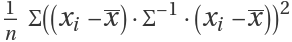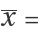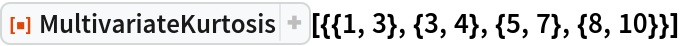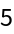Function Repository Resource:

# MultivariateKurtosis

Find the kurtosis coefficient for multivariate data

Contributed by: Wolfram Research
 ResourceFunction["MultivariateKurtosis"][matrix] gives a multivariate kurtosis coefficient for matrix.

## Details and Options

ResourceFunction["MultivariateKurtosis"] is a univariate measure of kurtosis for multivariate data.
ResourceFunction["MultivariateKurtosis"][matrix] is equivalent towhere matrix={x1,x2,,xn},Mean[matrix] and Σ is the estimated population covariance matrix.
For a matrix with p columns, a value of the multivariate kurtosis coefficient close to p (p+2) indicates approximate multinormality.

## Examples

### Basic Examples (1)

MultivariateKurtosis for bivariate data:

 In:=Out=## Requirements

Wolfram Language 11.3 (March 2018) or above

## Version History

• 1.0.0 – 20 February 2019